##Polynomial Long Division#### An Example.

In this section you will learn how to rewrite a rational function such as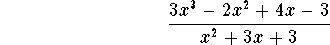in the form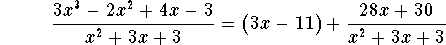The expression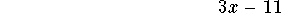is called the quotient, the expression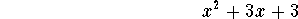is called the divisor and the term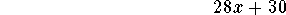is called the remainder. What is special about the way the expression above is written? The remainder 28x+30 has degree 1, and is thus less than the degree of the divisor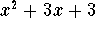.

It is always possible to rewrite a rational function in this manner:

 DIVISION ALGORITHM: If f(x) and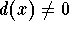are polynomials, and the degree of d(x) is less than or equal to the degree of f(x), then there exist unique polynomials q(x) and r(x), so that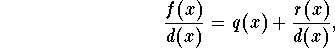and so that the degree of r(x) is less than the degree of d(x). In the special case where r(x)=0, we say that d(x) divides evenly into f(x).

How do you do this? Let's look at our examplein more detail. Write the expression in a form reminiscent of long division: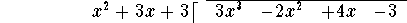First divide the leading term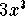of the numerator polynomial by the leading term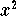of the divisor, and write the answer 3x on the top line: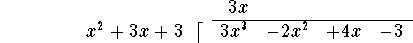Now multiply this term 3x by the divisor, and write the answer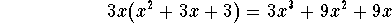under the numerator polynomial, lining up terms of equal degree: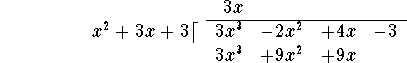Next subtract the last line from the line above it: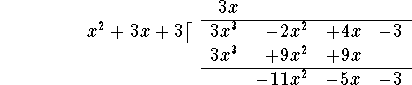Now repeat the procedure: Divide the leading term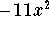of the polynomial on the last line by the leading termof the divisor to obtain -11, and add this term to the 3x on the top line: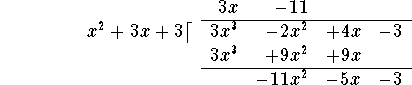Then multiply "back":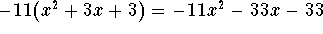and write the answer under the last line polynomial, lining up terms of equal degree: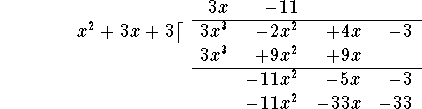Subtract the last line from the line above it: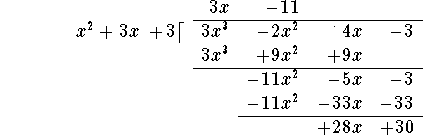You are done! (In the next step, you would divide 28x by, not yielding a polynomial expression!) The remainder is the last line: 28x+30, and the quotient is the expression on the very top: 3x-11. Consequently,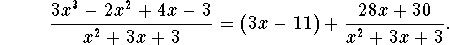The easiest way to check your answer algebraically is to multiply both sides by the divisor: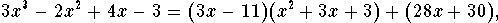then to multiply out: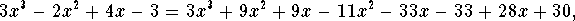and then to simplify the right side: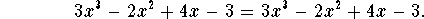Indeed, both sides are equal! Other ways of checking include graphing both sides (if you have a graphing calculator), or plugging in a few numbers on both sides (this is not always 100% foolproof).

#### Another Example.

Let's use polynomial long division to rewrite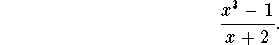Write the expression in a form reminiscent of long division: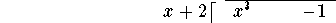First divide the leading term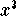of the numerator polynomial by the leading term x of the divisor, and write the answeron the top line: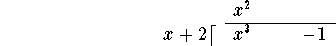Now multiply this termby the divisor x+2, and write the answer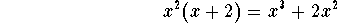under the numerator polynomial, carefully lining up terms of equal degree: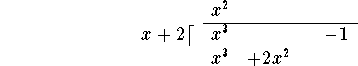Next subtract the last line from the line above it: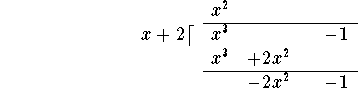Now repeat the procedure: Divide the leading term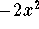of the polynomial on the last line by the leading term x of the divisor to obtain -2x, and add this term to theon the top line: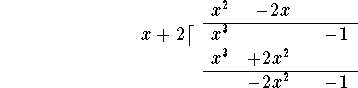Then multiply "back":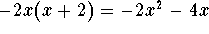and write the answer under the last line polynomial, lining up terms of equal degree: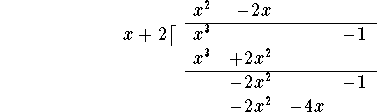Subtract the last line from the line above it: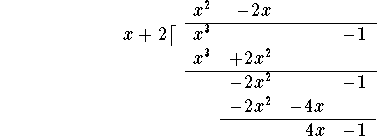You have to repeat the procedure one more time.

Divide: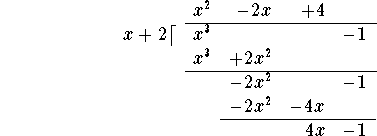Multiply "back":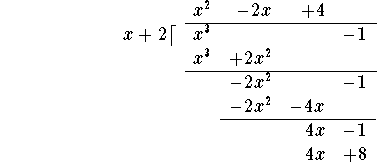and subtract: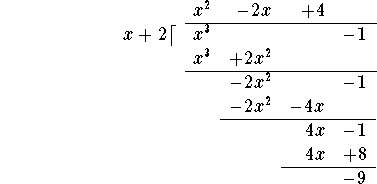You are done! (In the next step, you would divide -9 by x, not yielding a polynomial expression!) The remainder is the last line: -9 (of degree 0), and the quotient is the expression on the very top: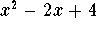. Consequently,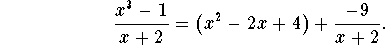#### An Example: Long Polynomial Division and Factoring.

Let's use polynomial long division to rewrite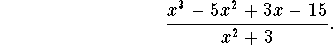Write the expression in a form reminiscent of long division: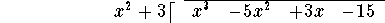First divide the leading termof the numerator polynomial by the leading termof the divisor, and write the answer x on the top line: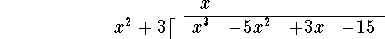Now multiply this term x by the divisor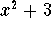, and write the answer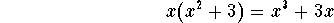under the numerator polynomial, carefully lining up terms of equal degree: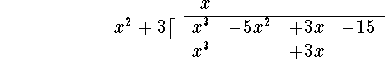Next subtract the last line from the line above it: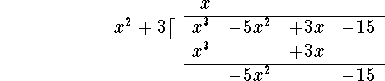Now repeat the procedure: Divide the leading term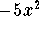of the polynomial on the last line by the leading termof the divisor to obtain -5, and add this term to the x on the top line: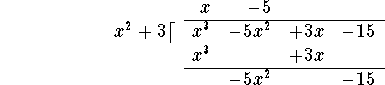Then multiply "back":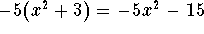and write the answer under the last line polynomial, lining up terms of equal degree: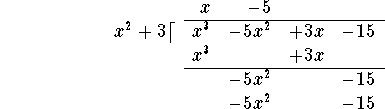Subtract the last line from the line above it: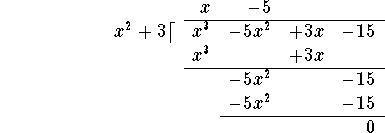You are done! In this case, the remainder is 0, sodivides evenly into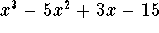.

Consequently,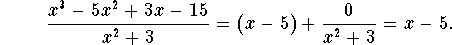Multiplying both sides by the divisoryields: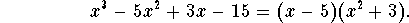In this case, we have factored the polynomial, i.e., we have written it as a product of two "easier" (=lower degree) polynomials.

#### Exercise 1.

Use long polynomial division to rewrite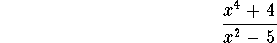#### Exercise 2.

Use long polynomial division to rewrite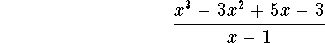#### Exercise 3.

Use long polynomial division to rewrite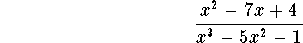#### Exercise 4.

Use long polynomial division to rewrite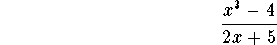#### Exercise 5.

Use long polynomial division to rewrite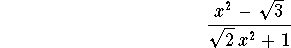[Back] [Next]
[Algebra] [Trigonometry] [Complex Variables]
[Calculus] [Differential Equations] [Matrix Algebra]S.O.S MATHematics home page

Do you need more help? Please post your question on our S.O.S. Mathematics CyberBoard.Helmut Knaust
Fri Jun 6 13:11:33 MDT 1997